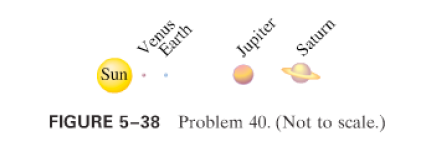×
Get Full Access to Physics: Principles With Applications - 6 Edition - Chapter 5 - Problem 40p
Get Full Access to Physics: Principles With Applications - 6 Edition - Chapter 5 - Problem 40p

×

# (II) Every few hundred years most of the planets line upISBN: 9780130606204 3

## Solution for problem 40P Chapter 5

Physics: Principles with Applications | 6th Edition

• Textbook Solutions
• 2901 Step-by-step solutions solved by professors and subject experts
• Get 24/7 help from StudySoup virtual teaching assistantsPhysics: Principles with Applications | 6th Edition

4 5 1 268 Reviews
26
5
Problem 40P

(II) Every few hundred years most of the planets line up on the same side of the Sun. Calculate the total force on the Earth due to Venus, Jupiter, and Saturn, assuming all four planets are in a line (Fig.). The masses are $$M_{V}=0.815 M_{E}, M_{j}=318 M_{E}, M_{s}=95.1 M_{E}$$, and their mean distances from the Sun are, and 1430 million, respectively. What fraction of the Sun's force on the Earth is this?Equation Transcription:Text Transcription:

MV=0.815ME, Mj=318ME, Ms=95.1ME

Step-by-Step Solution:

Step by step solution

Step 1

First we need to calculate the masses of planets in terms of Earth’s mass (ME)

Mass of VenusMass of JupiterMass of SaturnDistances of planets from the Sun:Step 2 of 6

Step 3 of 6

##### ISBN: 9780130606204

This textbook survival guide was created for the textbook: Physics: Principles with Applications, edition: 6. The full step-by-step solution to problem: 40P from chapter: 5 was answered by , our top Physics solution expert on 03/03/17, 03:53PM. The answer to “?(II) Every few hundred years most of the planets line up on the same side of the Sun. Calculate the total force on the Earth due to Venus, Jupiter, and Saturn, assuming all four planets are in a line (Fig. ). The masses are $$M_{V}=0.815 M_{E}, M_{j}=318 M_{E}, M_{s}=95.1 M_{E}$$, and their mean distances from the Sun are , and 1430 million , respectively. What fraction of the Sun's force on the Earth is this? Equation Transcription:Text Transcription:MV=0.815ME, Mj=318ME, Ms=95.1ME” is broken down into a number of easy to follow steps, and 80 words. Since the solution to 40P from 5 chapter was answered, more than 563 students have viewed the full step-by-step answer. This full solution covers the following key subjects: planets, sun, line, Force, EARTH. This expansive textbook survival guide covers 35 chapters, and 3914 solutions. Physics: Principles with Applications was written by and is associated to the ISBN: 9780130606204.

## Discover and learn what students are asking

Unlock Textbook Solution